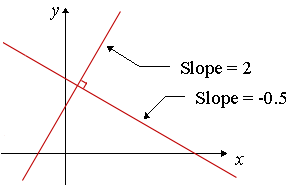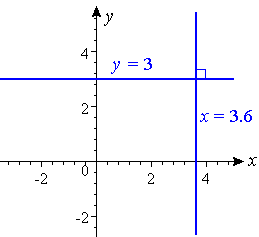Search IntMath
Close

450+ Math Lessons written by Math Professors and Teachers

5 Million+ Students Helped Each Year

1200+ Articles Written by Math Educators and Enthusiasts

Simplifying and Teaching Math for Over 23 Years

# Perpendicular Lines

Perpendicular lines have the property that the product of their slopes is −1.Mathematically, we say if a line has slope m1 and another line has slope m2 then the lines are perpendicular if

m1 × m2= −1

In the example at right, the slopes of the lines are 2 and -0.5 and we have:

2 × −0.5 = −1

So the lines are perpendicular.

## Opposite Reciprocal

Another way of finding the slope of a perpendicular line is to find the opposite reciprocal of the slope of the original line.

In plain English, this means turn the original slope upside down and take the negative.

## Interactive graph - perpendicular lines

You can explore the concept of perpendicular lines in the following JSXGraph (it's not a fixed image).

Drag any of the points A, B or C and observe the slopes m1, m2 of the 2 perpendicular lines.

You can move the graph up-down, left-right if you hold down the "Shift" key and then drag the graph.

Sometimes the labels overlap. It can't be helped!

If you get lost, you can always refresh the page.

### Special Cases

What if one of the lines is parallel to the y-axis?

For example, the line y = 3 is parallel to the x-axis and has slope 0. The line x = 3.6 is parallel to the y-axis and has an undefined slope.

The lines are clearly perpendicular, but we cannot find the product of their slopes. In such a case, we cannot draw a conclusion from the product of the slopes, but we can see immediately from the graph that the lines are perpendicular.The same situation occurs with the x- and y-axes. They are perpendicular, but we cannot calculate the product of the 2 slopes, since the slope of the y-axis is undefined.

### Exercise 1

A line L has slope m = 4.

a) What is the slope of a line parallel to L?

b) What is the slope of a line perpendicular to L?

a) Since parallel lines have the same slope, the slope will be 4.

b) Using m1 × m2= -1, with m1 = 4, we obtain the value for m2:

m_2=-1/(m_1)

=-1/4

### Exercise 2

A line passes through (-3, 9) and (4, 4). Another line passes through (9, -1) and (4, -8). Are the lines parallel or perpendicular?

The line through (-3,9) and (4,4) has slope

m_1=(4-9)/(4-(-3))=(-5)/7

The line through (9,-1) and (4,-8) has slope

m_2=(-8-(-1))/(4-9)=(-7)/(-5)=7/5

Now

m_1m_2=(-5)/7xx7/5=-1

Since the product of the slopes is −1, we conclude the lines are perpendicular.

Note: We could have sketched the lines to determine whether they were parallel or perpendicular.

## Problem SolverThis tool combines the power of mathematical computation engine that excels at solving mathematical formulas with the power of GPT large language models to parse and generate natural language. This creates math problem solver thats more accurate than ChatGPT, more flexible than a calculator, and faster answers than a human tutor. Learn More.